## Cipher## May 26, 2017

### CNN: 23 dead on a bus carrying Coptic Christians? +Palm Sunday Hoax

http://edition.cnn.com/2017/05/26/africa/egypt-shooting-coptic-christians/index.html
They just can't make these stories believable.. Especially when you see the numbers..

So on April 9th we saw Palm Sunday bombings in Egypt, and 47 days later we see another attack on Egypt?

Christian = 3+8+9+9+1+2+9+1+5 = 47 (Full Reduction)
gunmen = 7+21+14+13+5+14 = 74 (English Ordinal)
You already know how 47 and 74 are stamped all over Christianity = 3+8+9+9+10+2+9+1+5+9+2+7 = 74 (Single Reduction)100 kilometers? 62 miles?
sixty two = 10+9+6+2+7 + 2+5+6 = 47 (Single Reduction)
hundred = 8+21+14+4+18+5+4 = 74 (English Ordinal)

It took place near the city of Minya?
Egypt = 5+7+7+7+2 = 28 (Full Reduction)
Minya = 5+9+4+2+8 = 28 (Reverse Reduction)
Minya is located at 28° North
On Palm Sunday there were 28 people killed in Tanta supposedly

Elevation - 47 meters? a span of 47 days between these 2 events? Give me a break...
Minya = 30+9+40+400+1 = 480 (Jewish)
If we include the day of attack, this happened on the 48th day from Palm Sunday on a date with 48 numerology (5+26+17 = 48)

Palm Sunday = 16+1+12+13 + 19+21+14+4+1+25 = 126 (English Ordinal)
Note how there were 126 non-fatal injuries on that day...
Palm Sunday = 7+1+3+4 + 10+3+5+4+1+7 = 45 (Single Reduction)
45 dead?
Location: "Tanta and Alexandria" = 7+26+13+7+26 + 26+13+23 + 26+15+22+3+26+13+23+9+18+26 = 322 (Reverse Ordinal)
Classic case of bullshit propaganda.

We have some updates from CNN

Imagine they have 23 dead on a date with 23 numerology? (5+2+6+2+0+1+7 = 23)
4/9/2017 = 4+9+2+0+1+7 = 23 (Palm Sunday bombings)

May Twenty sixth = 4+1+7 + 2+5+5+5+2+7 + 10+9+6+2+8 = 73 (Single Reduction)
Egypt = 5+7+25+16+20 = 73 (English Ordinal)
The bus was on it's way to Saint Samuel monastery
Saint Samuel = 8+8+9+4+7 + 8+8+5+6+4+6 = 73 (Reverse Reduction)
State of emergency = 1+2+1+2+5 + 6+6 + 5+4+5+9+7+5+5+3+7 = 73 (Full Reduction)
Egypt "is still under a three month state of emergency period following twin attacks on Coptic churches"

According to Wikipedia and 2017 reports Egypt has about 93-95 million but they choose to report 91 million (13th triangular number is 91) - http://www.worldometers.info/world-population/egypt-population/
"Ninety one million" = 13+18+13+22+7+2 + 12+13+22 + 14+18+15+15+18+12+13 = 227 (Reverse Ordinal) the 49th prime number is 227 connecting to 4/9, Palm Sunday attacks
5/26/2017 = 5+26 +2+0+1+7 = 41 - 13th prime number
Palm Sunday bombing was on 4/9 = 13 and this one comes on 5/26 = 31?

On the Coptic Calendar new year starts on 9/11 (254th day of the Gregorian Calendar)
Coptic Calendar = 24+12+11+7+18+24 + 24+26+15+22+13+23+26+9 = 254 (Reverse Ordinal)
Coptic Christians = 3+15+16+20+9+3 + 3+8+18+9+19+20+9+1+14+19 = 186 (English Ordinal)Coptic Christians shot dead on bus = 6+3+2+7+9+6 + 6+1+9+9+8+7+9+8+4+8 + 8+1+3+7 + 5+4+8+5 + 3+4 + 7+6+8 = 171 (Reverse Reduction)
171st prime number is 1019

Coptic = 3+15+16+20+9+3 = 66 (English Ordinal)
From this "attack" to the new year on Coptic calendar is 3 months and 17 days (66th prime number is 317)

From Palm Sunday bombings to Coptic new year (9/11) is a 156 days...
The attack comes as the country is still under a three-month state of emergency period following twin attacks on Coptic churches on Palm Sunday last month that killed dozens of people, in attacks claimed by ISIS.
"Twin attacks" = 7+4+18+13 + 26+7+7+26+24+16+8 = 156 (Reverse Ordinal)
twin attacks = 2+5+9+5 + 1+2+2+1+3+2+1 = 33 (Full Reduction)
Coptic = 6+3+2+7+9+6 = 33 (Reverse Reduction)

CNN keeps bumping up the number of victims as usual, we're up to 26 nowMay 26th, 62 miles northwest of Minya, 26 people get shot and killed?Ten gunmen = 2+5+5 + 7+3+5+4+5+5 = 41 (Full Reduction)
5/26/2017 = 5+26+2+0+1+7 = 41

Another update, we had 25 injured and 26 dead, now we have 28 dead and 23 injured (23 injured goes well with a date numerology of 23)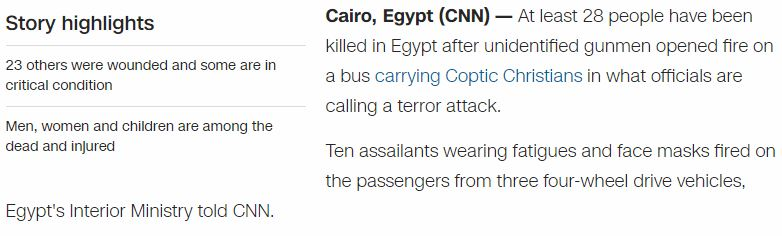They might leave the death count on 28, the 2nd perfect number, also it connects nicely to 33 and 156 (911) coding
twenty eight = 20+23+5+14+20+25 + 5+9+7+8+20 = 156 (English Ordinal)
thirty three = 20+8+9+18+20+25 + 20+8+18+5+5 = 156 (English Ordinal)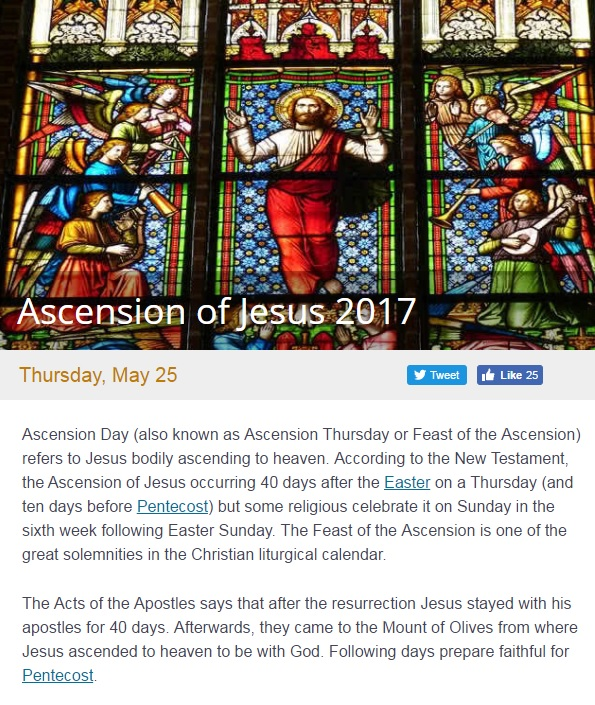This is very interesting, on May 25th Christians were celebrating Ascension of Jesus that comes 40 days after the Easter... and just the next day Christians get attacked on the way to monastery
Forty days = 3+3+9+7+2 + 5+8+2+8 = 47 (Reverse Reduction)

Also it's very interesting how we have 1 month and 26 days from Palm Sunday to Pentecost
Pentecost = 11+22+13+7+22+24+12+8+7 = 126 (Reverse Ordinal)
Palm Sunday = 16+1+12+13 + 19+21+14+4+1+25 = 126 (English Ordinal) - 126 injured
Coincidence? I don't think so

## May 19, 2017

### Quick Roulette Decode

Everybody knows that numbers on a Roulette wheel sum to 666 (36th triangular number), but there's a few more sixes to be foundIn the middle column we have 4 red numbers (5+14+23+32 = 74), and in the right column we have 3+9+12+18+21+27+30+36 = 156, both of these numbers appear frequently in combination with 33 (156th Fibonacci number is 33 digits long) - Thirty three = 20+8+9+18+20+25 + 20+8+18+5+5 = 156 (English Ordinal)
Six six six = 19+9+24 + 19+9+24 + 19+9+24 = 156 (English Ordinal)
Roulette board = 18+15+21+12+5+20+20+5 + 2+15+1+18+4 = 156 (English Ordinal)
Roulette table = 18+15+21+12+5+20+20+5 + 20+1+2+12+5 = 156 (English Ordinal)thirty two inches = 20+8+9+18+20+25 + 20+23+15 + 9+14+3+8+5+19 = 216 (English Ordinal)
32 inches is in fact 81.28 centimeters (4th perfect number is 8128!) also note that 32nd hexagonal number is 2016, on the Roulette wheel with 1 zero the first number to the right of it is 32If we take 1st and last row of the Roulette board they sum to 111, and so do 2nd and 11th, 3rd and 10th, 4th and 9th, 5th and 8th, 6th and 7th.... which reminds me a lot of the magic square of the Sun which encodes 666 in a 6x6 square... In the magic square of the Sun we have 2 main diagonals, rows and columns adding up to 111A white ball spinning around the wheel could stand for the Sun, also in the game of Poker white dealer button is rotated around the table in clockwise direction... If you ever sun gazed you would understand why they would use white instead of orange or some other color for the Sun.

## May 15, 2017

### Powers Boothe dies at 68 (Sin City, Agents of S.H.I.E.L.D.)

Quick decode on the death of Powers Boothe (or retirement), this one looks very suspicious, if he was killed it was planned for a while but at the moment I'm leaning towards retirement, he supposedly died of natural causes in his sleep at the age of 68Powers = 7+6+5+5+9+1 = 33 (Full Reduction) dies on Sunday = 8+6+4+5+8+2 = 33 (Reverse Reduction)
Boothe = 2+6+6+2+8+5 = 29 (Full Reduction) died on a date with 29 numerology (5+14+2+0+1+7)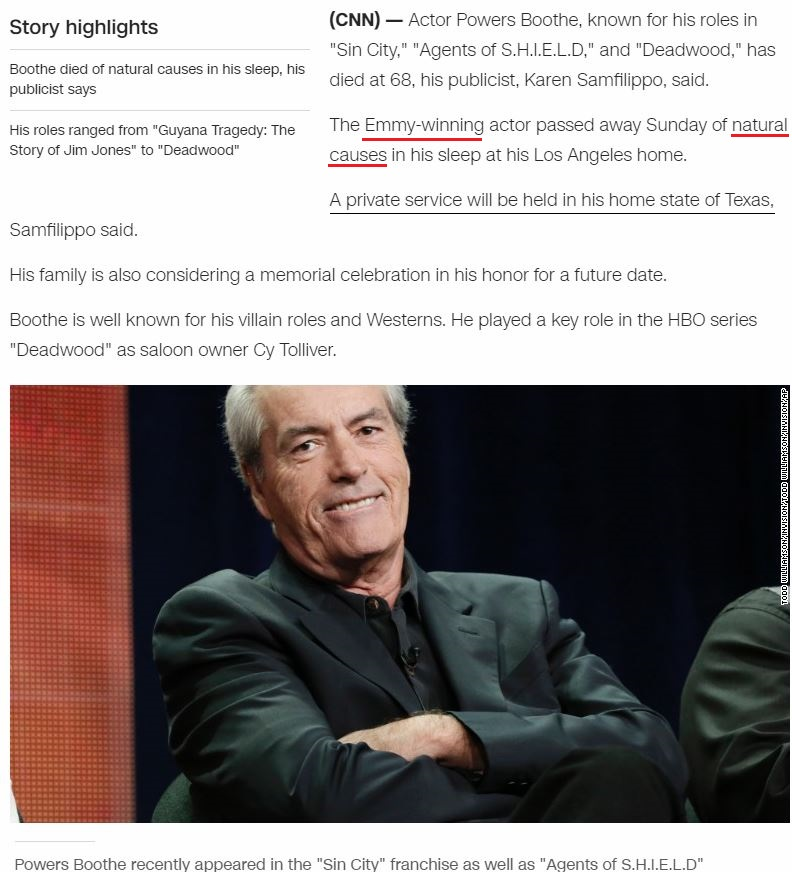There are very interesting connections to Sin City that is mentioned in this article, in the movie he plays the main antagonist called "Senator Roark" = 1+5+5+1+2+6+9 + 9+6+1+9+2 = 56 (Full Reduction) and he died at "Sixty eight" = 1+9+6+2+7 + 5+9+7+8+2 = 56 (Full Reduction) on a date with 56 numerology (5+14+20+17)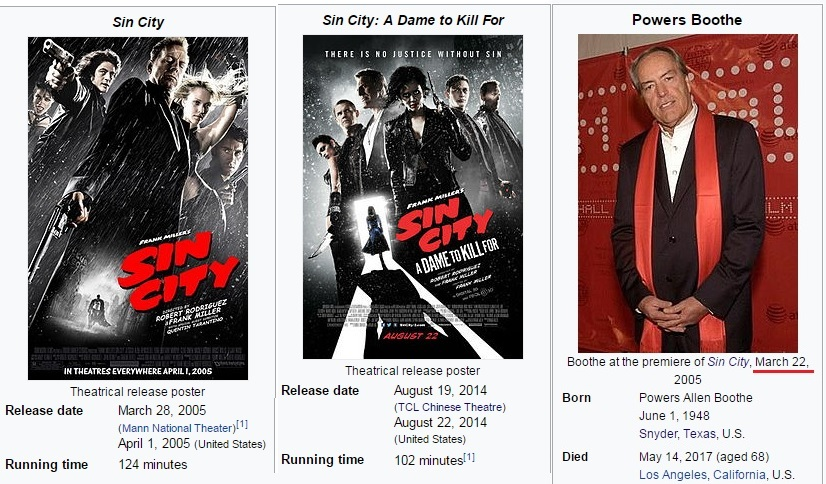Between Sin City movie releases we find 3430 days (7x7x7 = 343),  "Sin City" = 1+9+5 + 3+9+2+7 = 36 (Full Reduction) - Boothe died on a date with 36 numerology (5+14+17)From the release of Sin City: A Dame to Kill for on 8/22/2014 to Boothe's next birthday is 9 months and 11 daysMeasuring to his death from release of the sequel we find something even more interesting2 years 8 months 22 days from 8/22/2014, also some would say that 996 can be rearranged into 666, August 22nd is 234th day of the year (234+432 = 666), also they mention that Boothe was involved with "Agents of Shield" = 26+20+22+13+7+8 + 12+21 + 8+19+18+22+15+23 = 234 (Reverse Ordinal)

There's some 44 coding popping up and it goes well with "Agents of SHIELD" = 1+7+5+14+20+19 + 15+6 + 19+8+9+5+12+4 = 144 (English Ordinal)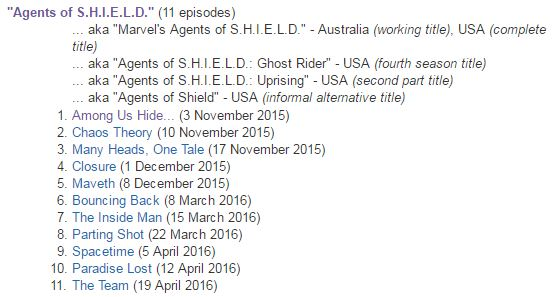He appeared on Agents of Shield for the last time on 4/19/2016, 44 days before his next birthday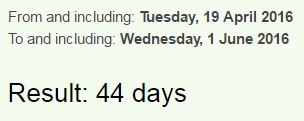Forty four = 6+15+18+20+25 + 6+15+21+18 = 144 (English Ordinal)
His birthday is also falling on a date with 44 numerology this year (6+1+20+17 = 44), Kill = 44 (English Ordinal)Execution 44 (Full Reduction)
Sin City: A Dame to Kill for came out on a date with 44 numerology as well (8+22+14)

CNN mentions that he's Emmy Winning actor and from his death to Emmy Awards this year we find 4 months and 4 days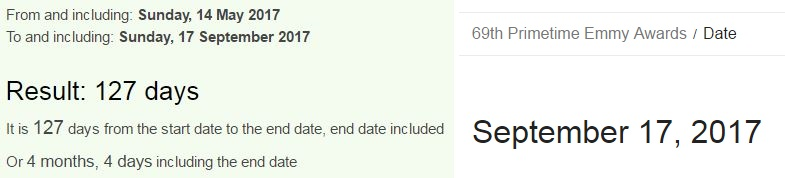Powers Boothe = 7+6+5+5+9+10 + 2+6+6+2+8+5 = 71 (Single Reduction)
44 and 71 are the only 2 numbers that sum to 144 when written out - Seventy one = 19+5+22+5+14+20+25 + 15+14+5 = 144 (English Ordinal), he was also starring in a TV show called "Deadwood" = 4+5+1+4+23+15+15+4 = 71 (English Ordinal)

## May 8, 2017

### Deck of cards (Calendar) and Poker terms

I played Poker for a couple of years and I'm not sure why I didn't think of gematrinating some of this stuff earlier but the game of Poker has some good numbers popping up

Out of 52 playing cards that are used today we can see a clear connection to 4 Suits of Tarot Decks but people can't exactly agree on which symbols correspond to the modern playing cards so I'll skip dealing with the Tarot to make things very simple here.

The first thing you notice when you look at a deck of cards is that you have 2 colors - Red = 9+4+5 = 18 (Reverse Reduction)Black = 7+6+8+6+7 = 34 (Reverse Reduction), so red+black = 18+34 = 52 cards, red cards stand for the hot months of the year and the black ones for cold
There are 52 weeks in a year and both "Earth" and "Card game" have matching gematria
Earth = 5+1+18+20+8 = 52 (English Ordinal) (25 full reduction)
card game = 3+1+18+4 + 7+1+13+5 = 52 (English Ordinal)
Poker = 2+3+7+4+9 = 25 (Reverse Reduction)
bluff = 7+6+6+3+3 = 25 (Reverse Reduction)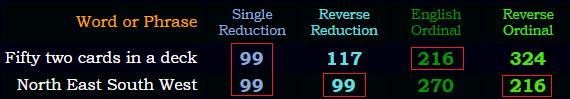The 2nd thing we see is 4 suits of 13 cards standing for "Four seasons" = 6+6+3+9 + 1+5+1+1+6+5+1 = 44 (Full Reduction), Deck of Cards = 4+5+3+2 + 6+6 + 3+1+9+4+1 = 44 (Full Reduction)Clock = 3+12+15+3+11 = 44 (English Ordinal) I find interesting that 52 is 44 in base 12 numbering system

In the deck we have 12 Face cards (Jacks, Queens, Kings) connecting to 12 months of the year, if you put each of the 12 face cards on each of the months and add up their values it sums to 144 - (11+12+13) x 4 seasons/suits = 144, Time = 100+9+30+5 = 144 (Jewish), Face cards = 6+1+3+5 + 3+1+80+4+90 = 193 (Jewish) <-- 44th prime number
13 cards in a suit is also connected to the Lunar Calendar

So here is how I laid out the cards over the calendar, it might not be 100% correct with the arrangement of the symbols I chose for the seasons but I was simply going with what made the most sense to me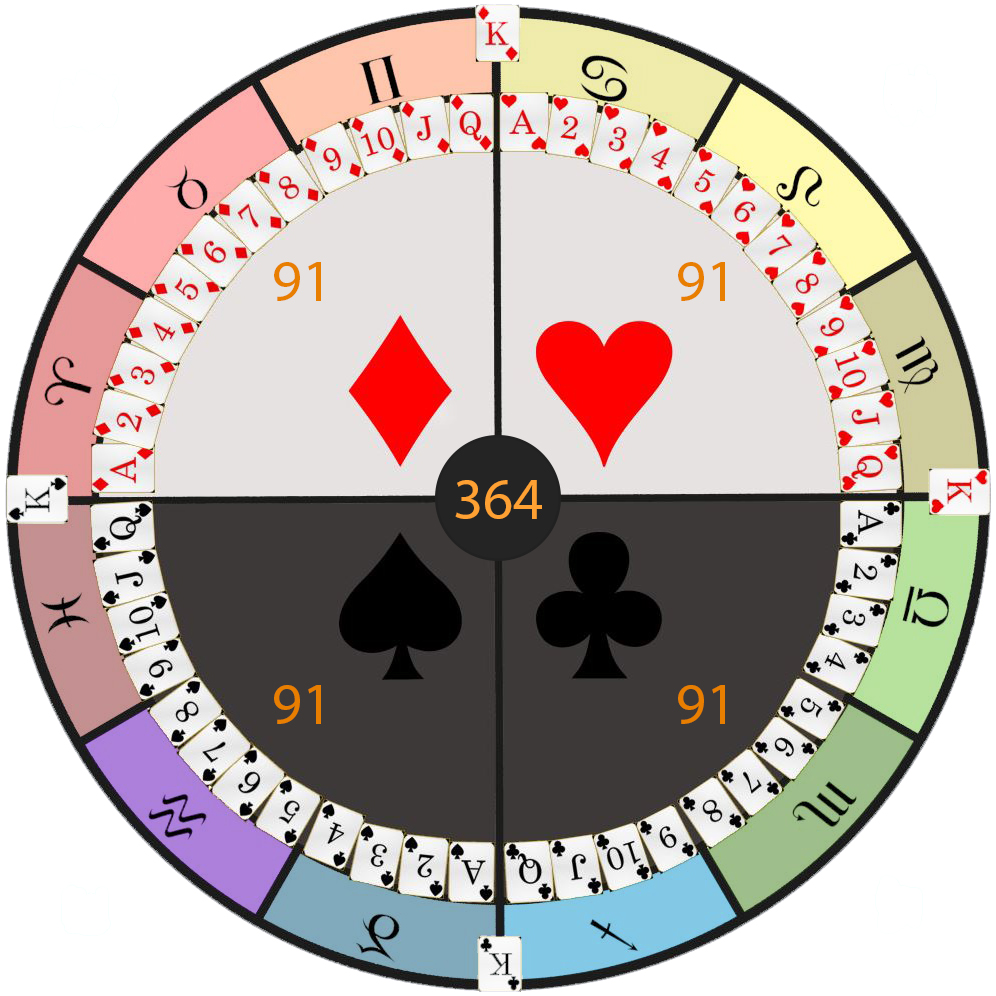So we have 4 suits of 13 cards and the 13th triangular number is 91 which is the length of a season in days (4x91 gives us 364 days of the year), Ninety one = 4+9+4+4+7+2 + 3+4+4 = 41 (Reverse Reduction), 13th triangular number written out sums to 41 (the 13th prime)
"Game of Poker" = 7+1+40+5 + 60+6 + 70+60+20+5+90 = 364 (English Extended)

Also it's important to notice that Kings fall on the 4 Cardinal points which is spelled "Kardinal" in many European languages, we also see 4 Kings mentioned in the Bible which encodes astrology all the way through

In the picture below you can see some correspondence between the opposing seasons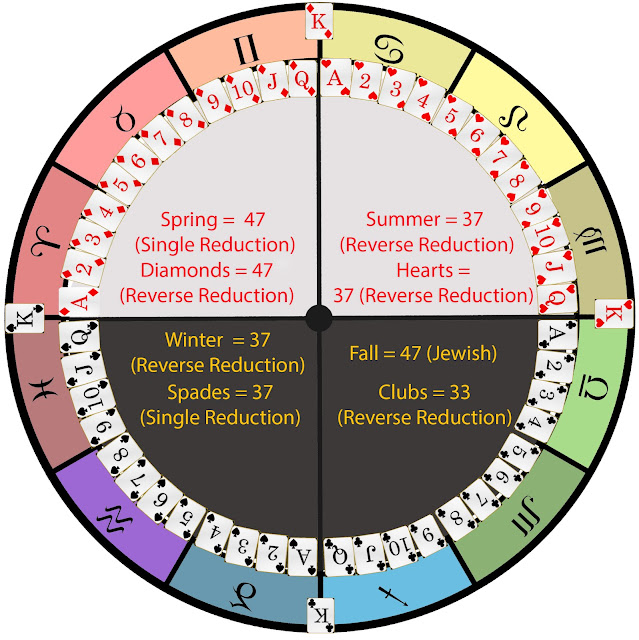Also Diamonds corresponds to Clubs through 33, Diamonds = 23+18+26+14+12+13+23+8 = 137 (Reverse Ordinal) <- 33rd prime number
Diamond = 4+9+1+4+6+5+4 = 33 (Full Reduction)

Here are some terms that come up when you're dealing with card games and mostly poker
All in = 8+6+6 + 9+4 = 33 (Reverse Reduction)
dealer button = 4+5+1+12+5+18 + 2+21+20+20+15+14 = 137 (English Ordinal)
"2" cards are also known as "Deuces" = 5+4+6+6+4+8 = 33 (Reverse Reduction)
"3" cards are also known as "Treys" = 2+9+5+7+10 = 33 (Single Reduction)
In Poker you can often hear "Two of a kind", "Three of a kind" or "Four of a kind" so if you leave out the number it also sums to 33 - "of a kind" = 6+6 + 1 + 2+9+5+4 = 33 (Full Reduction)
"Face cards" = 6+1+3+5 + 3+1+9+4+1 = 33 (Full Reduction)
"playing cards" = 7+3+1+7+9+5+7 + 3+1+9+4+10 = 66 (Single Reduction)
"Flush" = 6+12+21+19+8 = 66 (English Ordinal) - "Thirty three" = 2+8+9+9+2+7 + 2+8+9+5+5 = 66 (Full Reduction)
The strongest hand in Texas Hold'em is called "Royal flush" = 18+15+25+1+12 + 6+12+21+19+8 = 137 (English Ordinal)

"Poker Game" = 2+3+7+4+9 + 2+8+5+4 = 44 (Reverse Reduction)
"Pair" = 16+1+9+18 = 44 (English Ordinal)
"Call" = 3+1+20+20 = 44 (Jewish)
"Small Blind" = 10+4+1+3+3 + 2+3+9+5+4 = 44 (Single Reduction)

Another name for "Three of a kind" is "Triplets" = 20+18+9+16+12+5+20+19 = 119 (English Ordinal)
"Full house" = 6+21+12+12 + 8+15+21+19+5 = 119 (English Ordinal)
Here's something interesting connecting to WSOPNovember Nine? 11/9? Notice how "World Series of Poker" sums to 116 and you can easily flip that into 911
"World Series of Poker" = 5+6+9+3+4 + 10+5+9+9+5+10 + 6+6 + 7+6+2+5+9 = 116 (Single Reduction)
"Texas Hold'em" = 7+22+3+26+8 + 19+12+15+23 + 22+14 = 171 (Reverse Ordinal)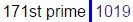## May 7, 2017

### Bobsled driver Steven Holcomb dies - sacrifice?

Steven Holcomb was found dead on May 6th 2017, there's very little information about his death right now but it definitely looks like a planned sacrificeSteven Holcomb was born on April 14th a date that would be written 4/14 in US and 14/4 in most parts of the world
Fourteenth of April = 6+60+300+90+200+5+5+50+200+8 + 60+6 + 1+70+90+9+30 = 1190 (English Extended)
He died on May 6th 2017
May sixth = 13+1+25 + 19+9+24+20+8 = 119 (English Ordinal)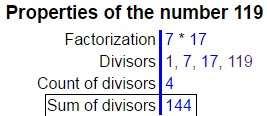Note that he was found dead in his room at the training center in Lake Placid = 12+1+11+5 + 16+12+1+3+9+4 = 74 (English Ordinal), died at 37 (74/2), Killing = 7444 connects to killing and 144 - Forty four = 6+15+18+20+25 + 6+15+21+18 = 144 (English Ordinal), notice how this connects with his birthday and the fact that he died in the sign of Taurus = 7+8+6+9+6+8 = 44 (Reverse Reduction)
He ended a 62 year drought in 2010 Winter Olympics and earned Gold Medal in four-man bobsled, "Gold Medal" = 2+3+6+5 + 5+4+5+8+6 = 44 (Reverse Reduction)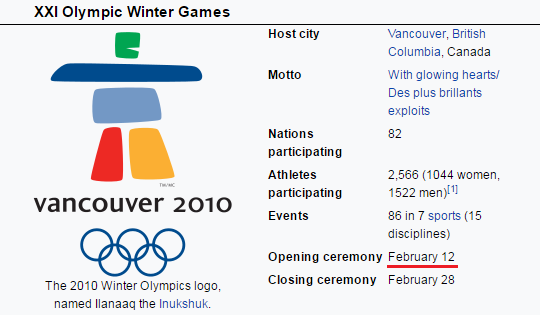Opening ceremony of the Olympic Winter games in 2010 came on a date with 44 numerology (2+12+20+10 = 44)
Bobsleigh = 2+50+2+90+20+5+9+7+8 = 193 (Jewish)
We have another connection to 44 in his place of birth (Park city = 70+1+90+20 + 3+9+200+700 = 1093 (English Extended), drop the zero and we are left with 44th prime number - 193)

Even the name of this website has 44 gematria.... http://www.teamholcomb.com/ - Team Holcomb = 2+5+1+4 + 8+6+3+3+6+4+2 = 44 (Full Reduction)

Honorable mention goes to CNN with their headline gematria as alwaysTeam USA bobsled driver Steven Holcomb dies = 153 (Full Reduction)
Steven Holcomb = 19+20+5+22+5+14 + 8+15+12+3+15+13+2 = 153 (English Ordinal)
Team USA bobsled driver Steven Holcomb dies = 216 (Reverse Reduction)
bobsled driver = 25+12+25+8+15+22+23 + 23+9+18+5+22+9 = 216 (Reverse Ordinal)
sixty two year drought = 8+18+3+7+2 + 7+4+12 + 2+22+26+9 + 23+9+12+6+20+19+7 = 216 (Reverse Ordinal)

Now here is the interesting part..Wikipedia says that he led the team to a gold medal victory on February 17th but all the news reports about the victory started coming in on the 27th and other Wikipedia links connecting to Bobsleigh competition talk about February 27th. I wonder if Wikipedia made honest mistake because 2/17 falls very interestingly with his time of death (measuring from 2/27/2010 to 5/6/2017 you find 7 years 2 months and 20 days)
February twenty seventh = 6+5+2+9+3+1+9+7 + 2+5+5+5+2+7 + 10+5+4+5+5+2+8 = 107 (Single Reduction)
Twenty two divided by seven = 2+5+5+5+2+7 + 2+5+6 + 4+9+4+9+4+5+4 + 2+7 + 1+5+4+5+5 = 107 (Full Reduction)We definitely find some 227 and connections to this competition
Olympic Winter Games = 15+12+25+13+16+9+3 + 23+9+14+20+5+18 + 7+1+13+5+19 = 227 (English Ordinal)
bobsled driver = 2+50+2+90+20+5+4 + 4+80+9+700+5+80 = 1051 (Jewish)If we measure from his last birthday to the time of his death we find 22 days but when we measure to his next birthday we find 343 days (octal of 227) or 344 days including the last date which is 11 months and 9 days..They chose a very special date to end this 62 year drought... and we have some 322's coming up as well
He died 22 days after his birthday - twenty two days = 200+500+5+50+200+700 + 200+500+60 + 4+1+700+100 = 3220 (English Extended)
Bobsleigh = 2+60+2+100+30+5+9+7+8 = 223 (English Extended)
sixty two years = 19+9+24+20+25 + 20+23+15 + 25+5+1+18+19 = 223 (English Ordinal)
Ending Bobsleigh drought after 62 years seems like we had some Skull and crossbones(223 Ordinal) involvement here, or maybe you could also call them "The synagogue of Satan" = 223 (English Ordinal)

From his birthday in 2009 until the end of the drought on 2/27/2010 is 320 daysNote that American team broke the drought on a date with 32 numerology (2+27+2+0+1+0 = 32) - America = 1+4+5+9+9+3+1 = 32 (Full Reduction)Bobsled = 2+6+2+10+3+5+4 = 32 (Single Reduction)Steven = 8+7+4+5+4+4 = 32 (Reverse Reduction)Holcomb = 8+6+3+3+6+4+2 = 32 (Single Reduction)
It's quite interesting how we have 32 date numerology on a date that encodes Pi (22/7 = 3.14) - Circle = 3+9+9+3+3+5 = 32 (Full Reduction), there are many parallels between Circle and America